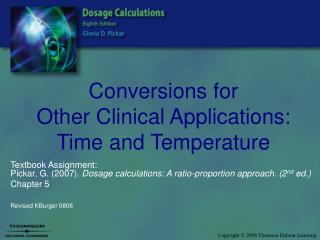DownloadDownload PresentationConversions for Other Clinical Applications: Time and Temperature

# Conversions for Other Clinical Applications: Time and Temperature

Télécharger la présentation## Conversions for Other Clinical Applications: Time and Temperature

- - - - - - - - - - - - - - - - - - - - - - - - - - - E N D - - - - - - - - - - - - - - - - - - - - - - - - - - -
##### Presentation Transcript

1. Conversions forOther Clinical Applications: Time and Temperature Textbook Assignment:Pickar, G. (2007). Dosage calculations: A ratio-proportion approach. (2nd ed.) Chapter 5 Revised KBurger 0808

2. Time • Time is an essential part of the drug order. • Traditional versus International (military) time. • What are some issues related to using the traditional time format? • What are the benefits of using International time format?

3. 24-Hour Clock

5. 3:15 p.m. 4:45 a.m. 5:30 p.m. 10:10 p.m. 12:35 a.m. What Time Is It?Convert to International Time 1515 0445 1730 2210 0035

6. What Is Wrong? • Give two Tylenol at 9:00 • Blood pressure to be taken at 2510 • Insulin given at 23:10 p.m. ??am or pm?? Beyond 24 hrs pm not necessary

7. TemperatureCelsius and Fahrenheit Scales • To convert between Fahrenheit and Celsius temperatures use the formula:

8. Celsius and Fahrenheit Scales

9. Celsius and Fahrenheit Scales • Using the FORMULA to:Convert 35˚ C to Fahrenheit F = [1.8 x 35]+ 32 F = 63 + 32 F = 95

10. Celsius and Fahrenheit Scales • Use the FORMULA to:Convert 102 F to Celsius 102 = [1.8 x C]+ 32 102-32 = [1.8 x C] +32 – 32 70 = 1.8C 70 = C1.8 38.9 = C

11. Calculate the Temperatures • 99˚ Fahrenheit • 75˚ Fahrenheit • 18˚ Celsius • 65˚ Celsius 37.2 Celsius 23.9 Celsius 64.4 Fahrenheit 149 Fahrenheit